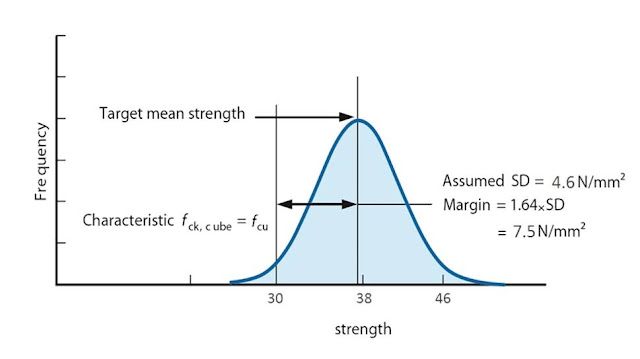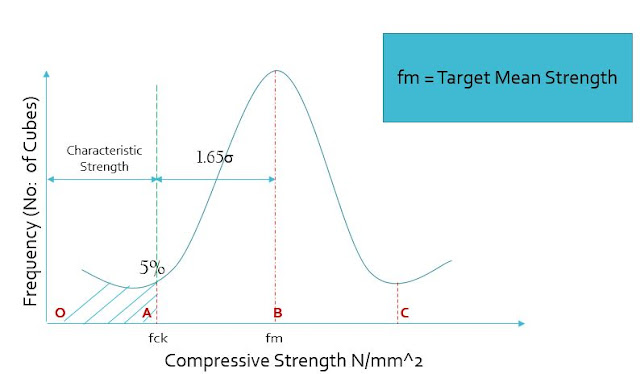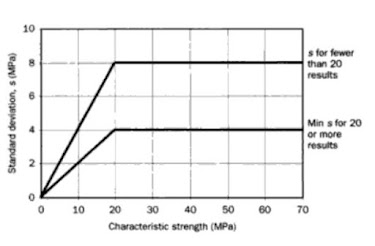### What is Target Mean Strength? R.C.C Design

Target mean strength (fm) is defined as the design strength determined for the manufacture of reinforced concrete. Target mean strength is determined by the formula:

fm = fck + 1.65𝛔

Where, fm = Target mean strength, fck = characteristic compressive strength, 𝛔 = standard deviationFig.1. Normal distribution curve  showing characteristic compressive strength and target mean strength, and margin.

In simple words, an M20 concrete implies a concrete with characteristic compressive strength of 20N/mm2. But in real practice, in order to make a concrete with a strength of minimum 20 N/mm2, we need to design a concrete with a higher value. Only then, after all possible losses if any, we finally get a concrete equal or greater than 20N/mm2. A higher strength value more than intended is fine, but a lesser value is not acceptable.

In the above equation, the quantity 1.65𝛔 represents the margin by which the target mean strength must exceed the required characteristic strength, and it is called as current margin. (Fig.1)

For Example:

If we need to design a concrete with characteristic mean strength of 30 N/mm2, what will be the target mean strength and the current margin to be used in the mix design, if standard deviation is 6 N/mm2?

Solution:

Target mean strength ,fcm = fck + 1.65𝛔 = 30 + (1.65 x 6 ) =  39.9 N/mm2
Current Margin                 = 9.9 N/mm2

### Standard Deviation and Current Margin in Target Mean Strength

After the analysis of numerous concrete test results, for various projects, the strength of concrete falls into some pattern of normal frequency distribution curve as shown in the figure-2 below.Fig.2. Normal Distribution Curve
The characteristic compressive strength is defined as the strength of the concrete below which not more than 5% of the test results are expected to fall.
The compressive strength of the cube samples although exhibit variations, when plotted on a histogram are found to follow the  a bell shape curve, as shown below.

This means, the area below fck is 5% of the total area and area after fck is 95 % of total area. This means 95 percent of the test results gave a value of strength greater than fck.

As the graph conveys, a failure of 5 % is normally chosen for concrete mix design and 95 percent of the samples must show a strength value greater than fck. This can be only possible if we, design the concrete using target mean strength.

If we analyze the standard deviation values for normal distribution curve, we get the distance between  A and B as 1.65𝛔. The mean of the curve is at B.

Characteristic strength is a lower limit of strength that is used in structural design. Concrete shows inherent variability of strength with all design mix materials, so we need to design the concrete for a mean strength value which is somewhat greater than the characteristic strength. This strength for which the concrete is design is the target mean strength. In the graph, the target mean strength is at B, which is OA + OB = fck + 1.65𝛔

The difference between the characteristic compressive strength and target mean strength = margin = 1.65𝛔

### Standard Deviation for R.C.C Mix Design

From the above explanation, it is clear that we need a clear idea on standard deviation to obtain the mean strength from a normal distribution curve. For an existing concrete production facility, previous test results will help. For areas with less previous results, a standard deviation versus characteristic strength of concrete graph can be referred, based on codes or regulations of the country.Fig.2. Standard Deviation V/S  Character Strength of Concrete ( BRE, 1997)

### Conclusion

Now from the graph, it is clear that the target mean strength increases with the increase in standard deviation. When there is poor quality control, higher standard deviation is used to obtain higher target mean strength  to obtain the desired strength.
Increasing the target mean strength would increase the cost of manufacture. If we really want to reduce the standard deviation, we need better quality control and hence higher cost. This hence demand a compromise.

In UK, the value of standard deviation is mainly between 4.5 to 7 N/mm2. A value less than 4 N/mm2 is seldom obtained.

This is why, quality of materials used in concrete mix design really matters the design strength.